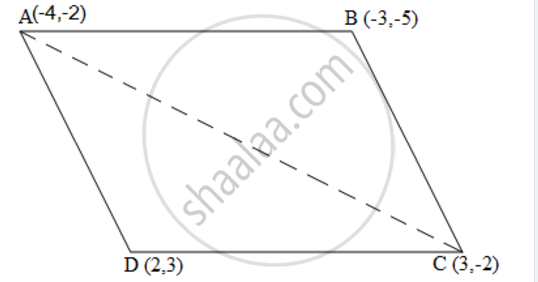# Find the Area of the Quadrilateral Whose Vertices, Taken in Order, Are (-4, -2), (-3, -5), (3, -2) and (2, 3). - Mathematics

Find the area of the quadrilateral whose vertices, taken in order, are (-4, -2), (-3, -5), (3, -2) and (2, 3).

#### SolutionLet the vertices of the quadrilateral be A ( - 4, - 2), B ( - 3, - 5), C (3, - 2), and D (2, 3). Join AC to form two triangles ΔABC and ΔACD.

Area of a triangle =1/2 {x_1 (y_2 - y_3) + x_2 (y_3 - y_1) + x_3 (y_1 - y_2)}

Area of ΔABC = 1/2 [(-4) {(-5) - (-2)} + (-3) {(-2) - (-2)} + 3 {(-2) - (-5)}]

= 1/2 (12+0+9)

= 21/2 square units

Area of ΔACD = 1/2 [(-4) {(-2) - (3)} + 3{(3) - (-2)} + 2 {(-2) - (-2)}]

= 1/2 (20+15+0)

= 35/2 square units

Area of ☐ABCD = Area of ΔABC + Area of ΔACD

=(21/2 + 35/2) square units = 28 square units

Concept: Area of a Triangle
Is there an error in this question or solution?
Chapter 7: Coordinate Geometry - Exercise 7.3 [Page 170]

#### APPEARS IN

NCERT Class 10 Maths
Chapter 7 Coordinate Geometry
Exercise 7.3 | Q 4 | Page 170
RD Sharma Class 10 Maths
Chapter 6 Co-Ordinate Geometry
Exercise 6.5 | Q 2.3 | Page 53
Share